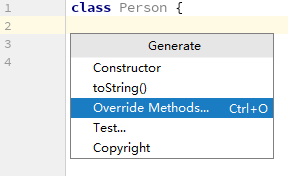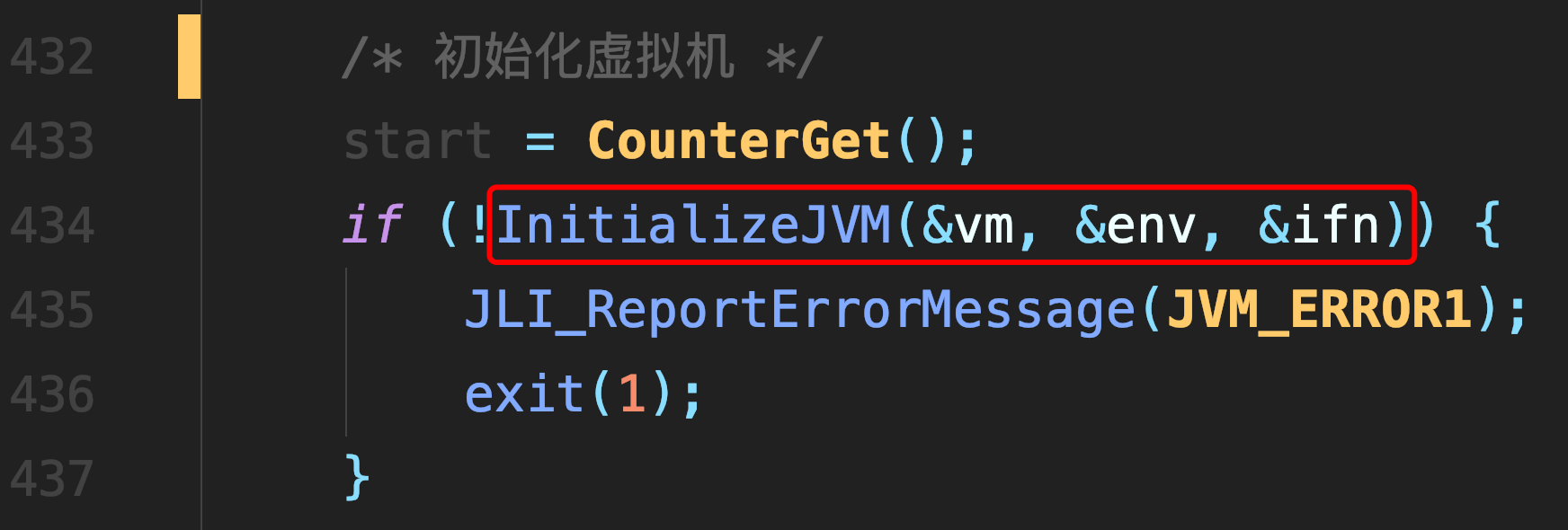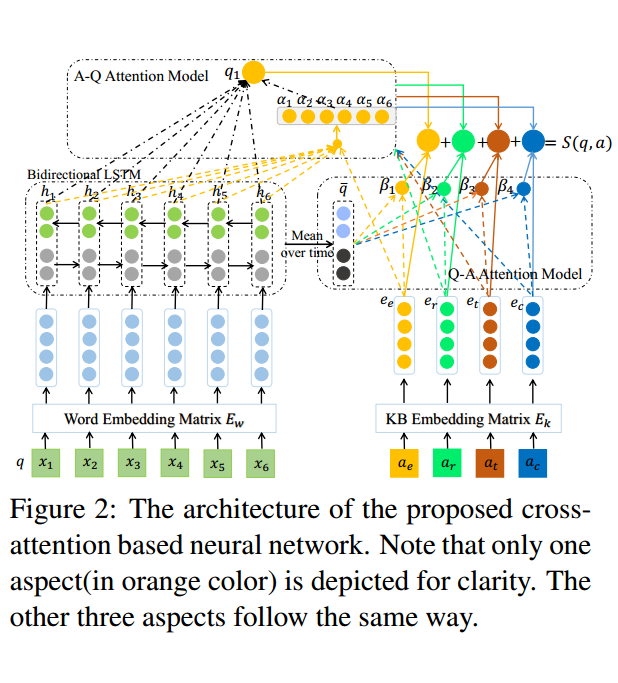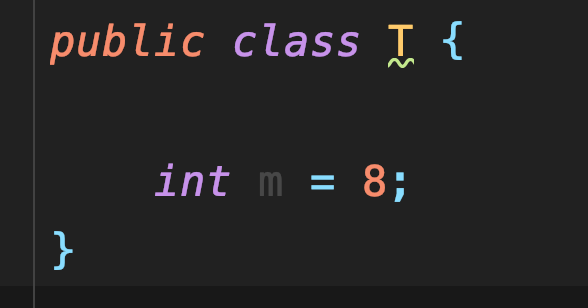# 前端中的Bootstrap以及 ECMAScript一些知识（原型链、闭包等）

### 1.Bootstrap

Bootstrap:响应式web,移动优先,前端组件库

HTML,CSS

样式、主题:按钮，表格，列表

封装了组件：导航，轮播，展板、对话框、进度条，卡片

组件： 预定义、可复用的功能模块

响应式：基于不同设备，提供不同的页面样式和布局（浏览器媒体查询，网格系统实现） viewport

分为5种：

``````xs  576 超小

sm  768 移动端

md  992 平板

lg  1200   桌面设备

xl  宽屏幕``````

网络系统 / 栅格系统    12列

Container： 1110 + 15 + 15

container-fluid：15,15

row

col-sm-1-12

Offset：偏移量

margin: 0 auto;：居中

### 2.ES

ECMAScript是JavaScript的核心，规定了JS的语法规范，是一种定义，标准。

Es6中let与var的区别：

``````1.let声明的变量是块级作用域(花括号括起来的那部分)，var声明是函数作用域和全局作用域

2.Let声明变量不会声明变量提升，var可以声明变量提升

3.Let不能声明同名变量，var可以声明``````

7 种数据类型：

``````            number

string

undefined

null

object

symbol

boolean

``````

null和 undefined的区别：

``````  1. 变量未赋值时，变量的值 undefined, 函数没有 return 语句, 则返回 undefined

2. null 是一个为 null 的值``````

``````                       null          undefined

number          0             NaN

string         'null'      'undefined'

boolean         false          false        ``````

### 3. 流程控制

DOM 操作  / jQuery   文档对象模型

HTML Document 树 ：

``````root

node 节点

Element

Text

Attr``````

（1）创建

``````       createElement('li')     独立的元素 追加到文档树：子元素或兄弟

append/appendChild      最后的子元素

prepend                 第一个子元素

before                  前一个兄弟

after                   后一个兄弟``````

（2）查找

``````          querySelector('css selector')       Element

querySelectorAll('css selector')    NodeList

nextSibling

previousSibling

parent``````

（3） 修改/替换

``    replace()  //label, input``

（4）删除

``  remove()``

### 4. 事件的绑定

（1） 静态绑定(html 的元素中增加 onXxx 属性事件 指定调用函数)

``````    <tag id="" class=""

οnclick="a()"

οnblur="b()"

onkey="

onmouse

style=""

>``````

（2） 动态绑定  JS

事件的冒泡:

ul

li

button

``````  e.addEventListener(

'事件',

() => {},

true

)

e.removeEventListener()

// 修改、增加样式（选择器

### 5.==与===的区别：

``````==是类型转化后比较值

===:比较严格，类型比较，类型相同才比较值``````

### 6.掩码

Ip:192.168.1.23

Mark:255.255.255.0

``````所有者   同组  其他

wxr      w--   --- 读写执行

111       100   000

7         4      0``````

### 7.位运算

1. & 与
``````1010

1100

——

1000``````

2.| 或

``````1010

1100

——

1110``````

3.^ 异或

``````1010

1100

——

0110``````

4.~取反

``````011

011

——

000``````

~3=-4； ~0=-1；~（-4）=3；

### 8.Stack（栈）

Stack是Vector的子类，是一个标准的先进后出的栈。

``````1.push()是用来在数组末端添加项，并且返回添加后数组的长度；

2、pop()在数组末端移除项，返回移除的数组元素；

3、unshift()在数组前端添加项，并且返回添加后数组的长度；

4、shift()在数组的第一个项（前端）移除，返回移除的数组元素。
``````

### 9.交换两个数数值的三种方法

· （1）借助第三变量

``````Int a=10; int b=15;

Int temp=a;

a=b;

b=temp;``````

（2）加减运算

``````a=a+b;

b=a-b;

a=a-b;``````

（3）异或运算

``````a=a^b;

b=a^b;

a=a^b;``````

### 10.判断一个数的奇偶（采用三元运算符）

``````// ? : (三元运算)

// a > b ? a : b

// n%2 == 0 ? '奇数' : '偶数'

// n&1 == 1 ? '奇数' : '偶数’``````

### 11.ES的一些变量

``````    let a;

typeof(a)

undefined   未定义

null        空

number      数值

string      字符串

boolean     逻辑

object      对象

symbol  标记/符号   ``````

### 12.   原型链

[],function,{}

原型链模型（继承）；

原型（模版）：

``````变量的 __proto__ 原型指向 它的 类型， 类型的原型属性执行父类型，  层层嵌套， 到上一级，最终都执行

Object，Object 的 __proto__ 是 null``````

### 13.闭包函数

``````   function f1() {

}

let f1 = function() {

}

let f1 = () => {

}

let x = f1;

x()

f1()``````

// 闭包: 函数和其中状态词法环境（上下文 context） 的结合构成闭包。

``````（1）内部函数可以持有并访问外部函数中变量的一种机 制，函数实例有状态。

（2）动态给多个元素注册事件监听器，回调函数需要传 递不同参数

（3）自定义组件，每个组件持有各自的状态 Vue

``````

`````` function add(x) {

let n = x;

return function(y) {

return n + y;

}

}

function abc(a, b) {

//

let x = a;

let y = b;

return {

a: function() {

},

b: function() {

}

}

}

let m1 = abc(3, 4);

let m2 = abc(100, 200);

m1.a()

m1.b()

m2.a()``````

##### 热门推荐
•浏览(612)
•浏览(390)
•浏览(365)
•浏览(351)
•浏览(347)
•浏览(310)
•浏览(292)
•浏览(281)
•浏览(267)
•浏览(251)